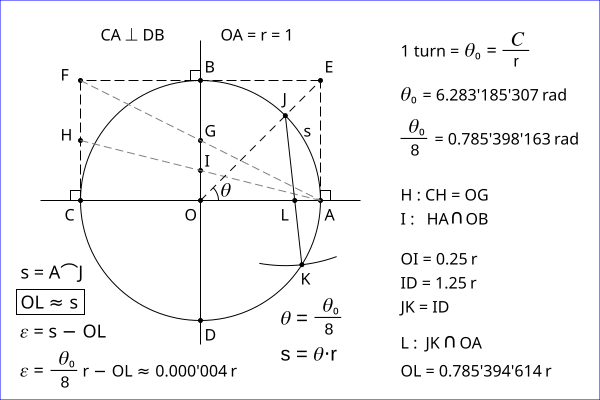# Squaring the circle

Although the number pi (𝜋 = C/D) can be used for calculations, I do not use it as a circle constant. The mathematical constant pi (𝜋) is by definition only a calculation number, and nothing else. But a turn has a physical meaning, and it is also a circle property. Thus, a turn is the circle constant. Please, see my article Circle constant is a turn.

Circle constant is the turn value in radian:

𝜃₀ = C/r ≈ 6.28 rad

Here are two methods of squaring the circle. You can use the button F11 for a full screen view.

The first method is based on the value 1 turn / 8 given by the Fig. 1a.Fig. 1a - Squaring the circle can be done by the arc length s of the angle 𝜃 = 1 turn / 8.

It is a simple method to find the arc length s of the angle 𝜃 = 1 turn / 8
(it is approximately the length OL). A turn is naturally the reference angle. Thus, the simple squaring the circle is given by the Fig. 1b.